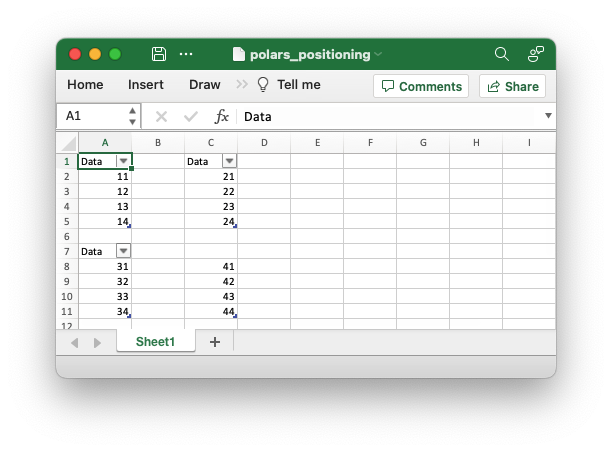# Example: Polars Excel dataframe positioning

An example of positioning dataframes in a worksheet using Polars and XlsxWriter. It also demonstrates how to write a dataframe without a header. See Working with Polars and XlsxWriter for more details.```##############################################################################
#
# An example of positioning dataframes in a worksheet using Polars and
# XlsxWriter.
#
# Copyright 2013-2023, John McNamara, jmcnamara@cpan.org
#

import xlsxwriter
import polars as pl

# Create some Polars dataframes from some data.
df1 = pl.DataFrame({"Data": [11, 12, 13, 14]})
df2 = pl.DataFrame({"Data": [21, 22, 23, 24]})
df3 = pl.DataFrame({"Data": [31, 32, 33, 34]})
df4 = pl.DataFrame({"Data": [41, 42, 43, 44]})

with xlsxwriter.Workbook("polars_positioning.xlsx") as workbook:
# Write the dataframe to the default worksheet and position: Sheet1!A1.
df1.write_excel(workbook=workbook)

# Write the dataframe using a cell string position.
df2.write_excel(workbook=workbook, worksheet="Sheet1", position="C1")

# Write the dataframe using a (row, col) tuple position.
df3.write_excel(workbook=workbook, worksheet="Sheet1", position=(6, 0))

# Write the dataframe without the header.
df4.write_excel(